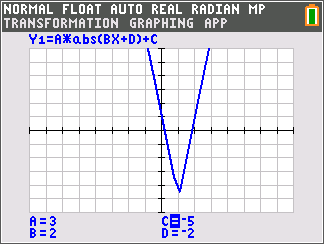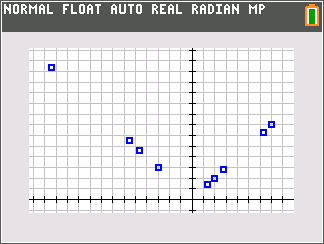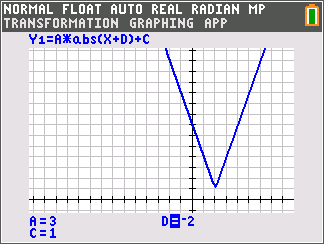•##### Download

•• ##### Device
• TI-84 Plus
• TI-84 Plus Silver Edition
•TI-84 Plus C Silver Edition
•TI-84 Plus CE
• ##### Software

TI Connect™ CE

• ##### Report an Issue

Algebra II: Introduction to Absolute Value
by Texas Instruments#### Overview

Students numerically explore absolute values using the calculator, plot points to graph y = |x|, and use the Transform application to perform transformations with absolute value functions.

#### Key Steps

•In the first part of this activity, students will use the graphing calculator to understand the concept of absolute value as the distance from zero. Then they will enter positive and negative x values into the list editor, which graphs the points.

•For the second part of the activity, students will investigate the effects of the variables A, C, and D on the graph of the function. They will focus on what determines the slopes of the sides and the placement of the vertex.

•There is an optional extension that explores the effects of the variables a, b, c, and d of the function y = a|bx + d| + c all on one graph.